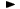ADVFN HomeHelpFinancialsDeeper AnalysisFINANCIAL RATIOS - Solvency RatiosTotal Debt/Pre-Tax Profit

# Total Debt/Pre-Tax Profit

Total Debt to Pre-Tax Profit ratio, is defined as total liabilities divided by pre-tax profit. The formula is the following:

total liabilities / pre-tax profit